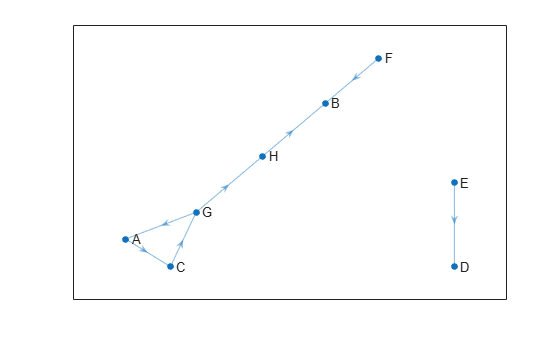# successors

Find successive state indices and costs

Since R2023a

## Syntax

``[succIDS,costs] = successors(graph,currID)``

## Description

example

````[succIDS,costs] = successors(graph,currID)` finds the state indices `succIDS` and the cost of all states `costs` that are connected to the specified state `currID` using the directed link.```

## Examples

collapse all

Load `navGraph` data for states and links.

`load navGraphData.mat`

Visualize the `navGraph` object.

`show(navGraphObj);`Find successive state indices and costs based on a state name.

`[succIDS,costs] = successors(navGraphObj,"G")`
```succIDS = 2×1 1 8 ```
```costs = 2×1 38.7759 13.9383 ```

Find the state vectors of the successive states.

`states = index2state(navGraphObj,succIDS)`
```states = 2×3 8.0000 2.0000 0.7218 8.0000 7.0000 0.6741 ```

## Input Arguments

collapse all

Graph object, specified as a `navGraph` object.

Current state, specified as a positive integer, representing a state index, or as a string scalar or character vector representing a state name.

Data Types: `double` | `char` | `string`

## Output Arguments

collapse all

Successive state indices, returned as a column vector of positive integers.

Data Types: `double`

Cost of all successive states, returned as a numeric column vector of the same size as `succIDS`. The function computes costs using the `Weight` or `LinkWeightFcn` property of the `navGraph` object `graph`.

Data Types: `double`

## Version History

Introduced in R2023a